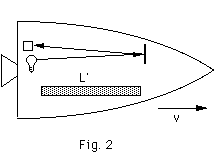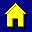Time Dilation

This is a derivation of the time dilation formula. This will be covered in detail in class, but here it is just in case it goes by too fast. Refer to Fig. 1.

A clock is made by sending a pulse of light toward a mirror at a distance L and back to a receiver. Each "tick" is a round-trip to the mirror. The clock is shown at rest in the "Lab" frame in Fig. 1a, or any time it is in its own rest frame. Consequently, it also represents the clock at rest in rocket#1. Figure 1b is the way the clock looks in the lab when the clock is at rest in rocket#1, which is moving to the right with velocity v.Actually, our clock "ticks" once every round-trip of the light pulse. So, we should use ct=2L, etc. But since both legs of the round-trip are the same, we'll just use the one-way times for simplicity.

Some notation:

t = time for light to reach the mirror in the lab for a clock at rest in Rocket #1 (Fig. 1b)
t' = time for light to reach the mirror in Rocket#1 in its own rest frame (Fig. 1a)
L = distance to mirror

So, the times and distances are related as follows:
L = ct'
L2 + v2*t2 = c2*t2 (Pythagorean theorem)

Eliminate L from the equations:
c2*t'2 = c2*t2 - v2*t2
t' = t*√(1-v2/c2)

Since √(1-v2/c2)<1, the clock at rest in rocket#1 appears slow to observers in the lab.

Go back to Fig. 1c. Rocket#2 is moving with velocity v to the left . Those clocks would also appear slow to observers in the lab. But, it is also the way a clock at rest in the lab appears in rocket#1. So, lab clocks also appear slow to observers in the rockets. Relativity is symmetrical!

Lorentz Contraction

This is a derivation of the Lorentz-contraction formula. We will go over the ideas in class, but the algebra is a bit gruesome. So, I'm putting that part here. Refer to Fig. 2.

As with the time dilation example, imagine a pulse of light reflects from a mirror back to a receiver. Light will be the "yardstick" used to measure the length of an object in the lab and rocket frames.Some notation:

L' = length of stick in the "rocket"
L = length of stick in the "lab"
t1 = travel time of light pulse to the end of the stick (lab)
t2 = travel time of light pulse back from the end of the stick (lab)
t = t1+t2 = total travel time in the lab
t' = total travel time in the rocket
v = speed of rocket in the lab frame

In the lab:
L + v*t1 = c*t1
L - v*t2 = c*t2
t = t1 + t2 = (2L/c)/(1-v2/c2)

In the rocket:
2L' = c*t'
t' = 2L'/c

We know from the time dilation formula that
t' = t*√(1-v2/c2)

So,
2L'/c = (2L/c)/√(1-v2/c2)
L' = L/√(1-v2/c2)
L = L'*√(1-v2/c2)

The stick appears shorter in the lab frame since √(1-v2/c2)<1.Return to the home page.